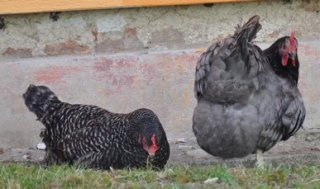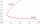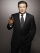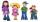# Chickens and rabbits

In the yard were chickens and rabbits. Together they had 27 heads and 86 legs. How many chickens and how many rabbits were in the yard?

Correct result:

chickens:  11
rabbits:  16

#### Solution:

a+b = 27
2•a+4•b=86

a+b = 27
2a+4b = 86

a = 11
b = 16

Our linear equations calculator calculates it.We would be pleased if you find an error in the word problem, spelling mistakes, or inaccuracies and send it to us. Thank you!Math student
We know that chicken has 2 legs and rabbit has 4. Assume that all heads are of chicken = 44*2 = 88. Now the difference between the total no. Of legs = 124-88 = 36. 36/2 =18 legs of Rabbits and 44-18 = 26 for chicken

2 years ago  21 LikesMath student
Imagine all rabbits and chickens are well trained. We blow the whistle, all chickens and rabbits would retract one of their legs. 124 - 44 = 80. Then we blow the whistle again, they retract another leg. 80-44= 36. these remaining 36 legs belong to the rabbits which are standing with two legs (the other two are retracted). thus 36/2 = 18 rabbits. and the chickens are 44-18 = 26.

2 years ago  6 LikesMath student
viju has 40 chickens and rabbits.  If in all there are 90 legs.  How many rabbits are there with viju??

4 years ago  11 LikesManikanta
35 chicken 5 rabbit

3 years ago  6 LikesMath student
Imagine all rabbits and chickens are well trained. We blow the whistle, all chickens and rabbits would retract one of their legs. 124 - 44 = 80. Then we blow the whistle again, they retract another leg. 80-44= 36. these remaining 36 legs belong to the rabbits which are standing with two legs (the other two are retracted). thus 36/2 = 18 rabbits. and the chickens are 44-18 = 26.

2 years ago  6 LikesMath student
We know that chicken has 2 legs and rabbit has 4. Assume that all heads are of chicken = 44*2 = 88. Now the difference between the total no. Of legs = 124-88 = 36. 36/2 =18 legs of Rabbits and 44-18 = 26 for chicken

2 years ago  21 LikesMath student
35 chiken and 5 rabit

2 years ago  3 LikesMath student
Nonsense question is something answering something elseMath student
40 chickens means 80 legs rest 10 legs .rabbit has 4 legs or 2 legs?10 legs will be shared among rabbit.

1 year ago  2 LikesTips to related online calculators
Do you have a linear equation or system of equations and looking for its solution? Or do you have quadratic equation?

## Next similar math problems:

• Fifth of the numberThe fifth of the number is by 24 less than that number. What is the number?
• The largerThe larger of two numbers is nine more than four times the smaller number. The sum of the two numbers is fifty-nine. Find the two numbers.
• EquationsSolve following system of equations: 6(x+7)+4(y-5)=12 2(x+y)-3(-2x+4y)=-44
• Hotel roomsIn the 45 rooms, there were 169 guests, some rooms were three-bedrooms and some five-bedrooms. How many rooms were?
• SchoolsThree schools are attended by 678 pupils. To the first attend 21 students more and to the third 108 fewer students than to second school. How many students attend the schools?
• The differenceThe difference of two numbers is 1375. If their exact quotient is 12. Find the two numbers
• Belgium vs ItalyBelgium played a match with Italy and Belgium win by 2 goals. The match fell a total 6 goals. Determine the number of goals scored by Belgium and by Italy.
• Three daysDuring the three days sold in stationery 1490 workbooks. The first day sold about workbooks more than third day. The second day 190 workbooks sold less than third day. How many workbooks sold during each day?
• Three friendsThe three friends spent 600 KC in a teahouse. Thomas paid twice as much as Paul. Paul a half less than Zdeněk. How many each paid?
• TheatroTheatrical performance was attended by 480 spectators. Women were in the audience 40 more than men and children 60 less than half of adult spectators. How many men, women and children attended a theater performance?
• Mom and daughterMother is 39 years old. Her daughter is 15 years. For many years will mother be four times older than the daughter?
• Football seasonDalibor and Adam together scored 97 goals in the season. Adam scored 9 goals more than Dalibor. How many goals scored each?
• ButtonsMen's shirt has sewn 9 buttons and blouse 3 buttons. Together they sewed 60 pieces and consumed 390 buttons. How many shirts?
• Dining roomThe dining room has 11 tables (six and eight seats). In total there are 78 seats in the dining room. How many are six-and eight-seat tables?
• Equations - simpleSolve system of linear equations: x-2y=6 3x+2y=4
• School yearAt the end of the school year has awarded 20% of the 250 children who attend school. Awat got 18% boys and 23% of girls. Determine how many boys and how many girls attend school.
• Two numbersThe difference between the two numbers is 74. If we divide a larger number by a smaller one, we get a quotient 7 and the rest of 2. Determine both numbers.